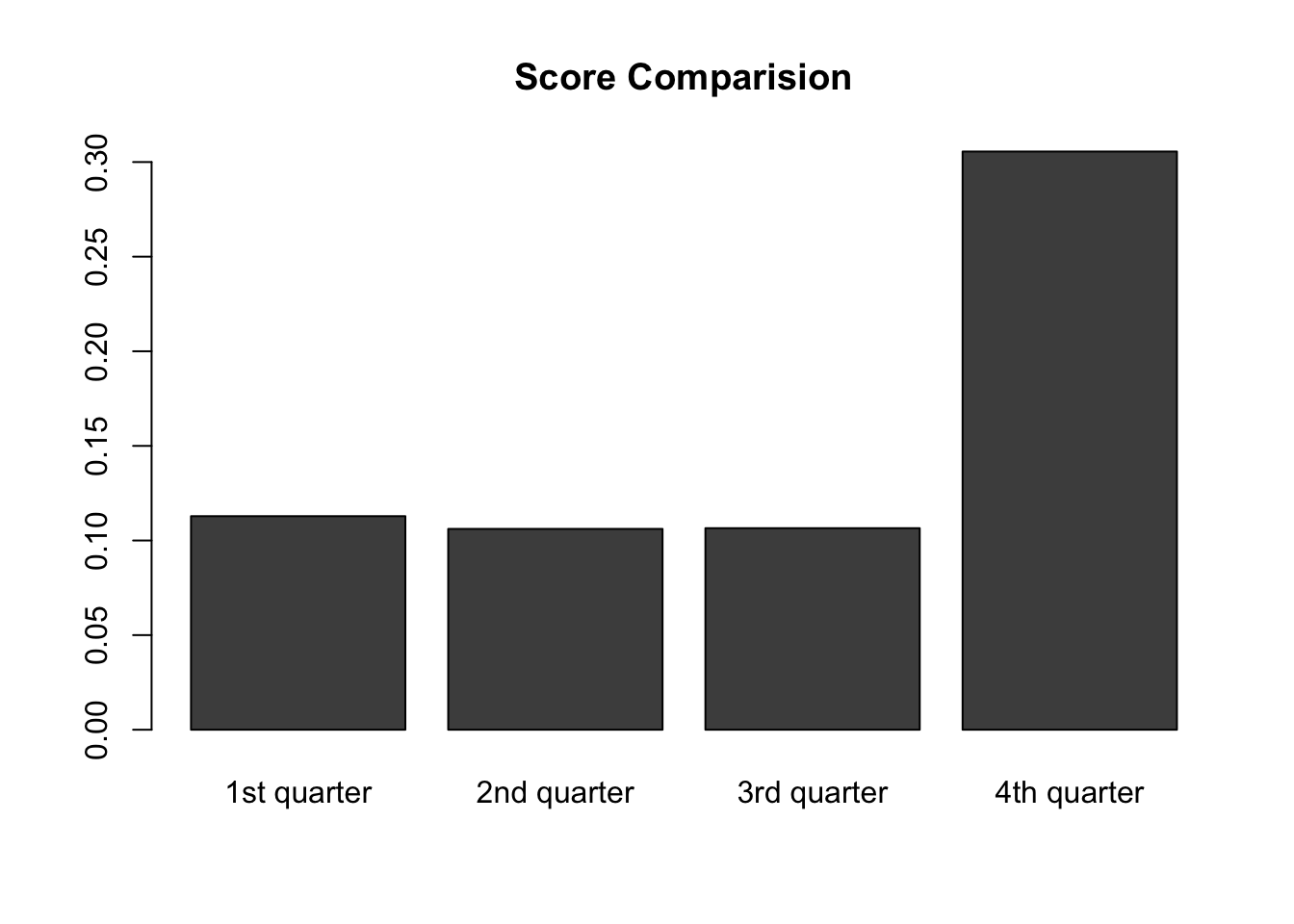library(tm)
## Loading required package: NLP
library(readr)

# Step 1 :Read in the AFINN word list and split the vector

AFINN <- read.delim("AFINN111.txt", header=FALSE)
# change column names to "Word" and "Score"
colnames(AFINN) <- c("Word","Score")

# Step 2:Compute the overall score for the MLK speech

 # read in text file MLK.txt
mlk <- mlk[which(mlk != "")]

# interprets each element of the "mlk" as a document and create a vector source
words.vec <- VectorSource(mlk)
# create a Corpus, a "Bag of Words"
words.corpus <- Corpus(words.vec)

# first step transformation: make all of the letters in "words.corpus" lowercase
words.corpus <- tm_map(words.corpus, content_transformer(tolower))
# second step transformation: remove the punctuation in "words.corpus"
words.corpus <- tm_map(words.corpus, removePunctuation)
# third step transformation: remove numbers in "words.corpus"
words.corpus <- tm_map(words.corpus, removeNumbers)
# final step transformation: take out the "stop" words, such as "the", "a" and "at"
words.corpus <- tm_map(words.corpus, removeWords, stopwords("english"))

# create a term-document matrix "tdm"
tdm <- TermDocumentMatrix(words.corpus)
# convert tdm into a matrix called "m"
m <- as.matrix(tdm)
# create a list of counts for each word named "wordCounts"
wordCounts <- rowSums(m)
# sort words in "wordCounts" by frequency
wordCounts <- sort(wordCounts, decreasing=TRUE)
# check the first ten items in "wordCounts" to see if it is built correctly
head(wordCounts, 10)
##    will freedom   negro     one     let    ring     day   dream  nation
##      26      20      13      13      13      12      11      11      10
##    come
##      10
  # calculate the total number of words
totalWords <- sum(wordCounts)
# create a vector that contains all the words in "wordCounts"
words <- names(wordCounts)

# locate which words in mlk speech appeared in AFINN word list
# returns 0 if one "mlk" word does not appeared in AFINN list
matched <- match(words, AFINN$Word, nomatch = 0) # calculate the matched words counts mCounts <- wordCounts[which(matched != 0)] # create a new dataframe that contains matched words and their counts, set ordinal numbers as row names match <- data.frame(names(mCounts), mCounts, row.names = c(1:length(mCounts))) # change column names to "word" and "counts" colnames(match) <- c("word","counts") # join the dataframe "match" with "AFINN" by "word" column in match and "Word" column in AFINN mergedTable <- merge(match, AFINN, by.x = "word" ,by.y = "Word") # calculate the overall score OverallScore <- sum(mergedTable$counts * mergedTable$Score)/totalWords # The overall score is 0.1343639 OverallScore ##  0.1343639 # Step 3: compute the sentiment score for each quarter # create a function to calculate scores for each quater myfunction <- function(q){ # interprets each element of the "mlk" as a document and create a vector source words.vec <- VectorSource(mlk) # create a Corpus, which is a "Bag of Words" words.corpus <- Corpus(words.vec) # define "cutpoint_l" as the first cut points; round the number to get an interger cutpoint_l <- round(length(words.corpus)*(q-1)/4) + 1 # define "cutpoint_r" as the second cut points; round the number to get an interger cutpoint_r <- round(length(words.corpus)*q/4) # create a word corpus for for each quarter (cut by cutpoints) words.corpus <- words.corpus[cutpoint_l: cutpoint_r] # word corpora transformation words.corpus <- tm_map(words.corpus, content_transformer(tolower)) words.corpus <- tm_map(words.corpus, removePunctuation) words.corpus <- tm_map(words.corpus, removeNumbers) words.corpus <- tm_map(words.corpus, removeWords, stopwords("english")) # create term document matrix tdm <- TermDocumentMatrix(words.corpus) m <- as.matrix(tdm) # calculate a list of counts for each word wordCounts <- rowSums(m) wordCounts <- sort(wordCounts, decreasing=TRUE) # calculate total words totalWords <- sum(wordCounts) # locate the mlk words appeared in Afinn list words <- names(wordCounts) matched <- match(words, AFINN$Word, nomatch = 0)
mCounts <- wordCounts[which(matched != 0)]
match <- data.frame(names(mCounts),mCounts,row.names = c(1:length(mCounts)))
colnames(match)<-c("word","counts")
# merge matched words with Afinn scores
mergedTable <- merge(match, AFINN, by.x = "word" ,by.y = "Word")
# calculate the total score
Score <- sum(mergedTable$counts * mergedTable$Score)/totalWords
# return the results
return(Score)
}

# apply function to first quarter
Score1 <- myfunction(1)
# apply function to second quarter
Score2 <- myfunction(2)
# apply function to third quarter
Score3 <- myfunction(3)
# apply function to forth quarter
Score4 <- myfunction(4)

# Step 4 : plot the results via a bar chart

  # combine scores of four quarters into one dataframe
Scores <- cbind(Score1, Score2, Score3, Score4)
# create a bar plot for the four scores
barplot(Scores, names.arg = c("1st quarter","2nd quarter","3rd quarter","4th quarter"), main = "Score Comparision")